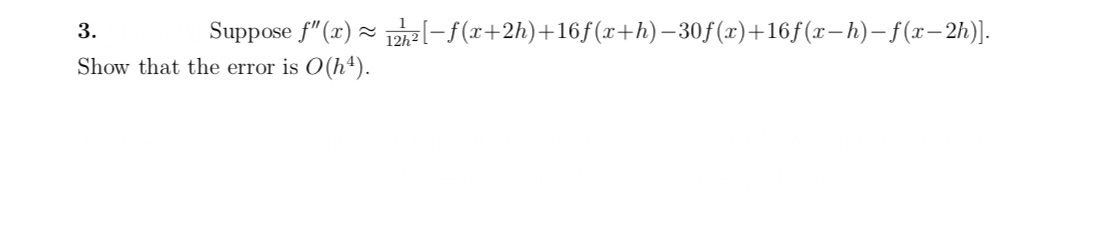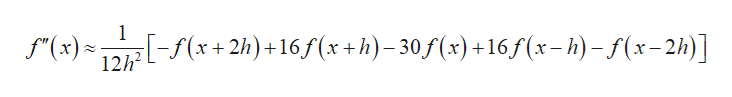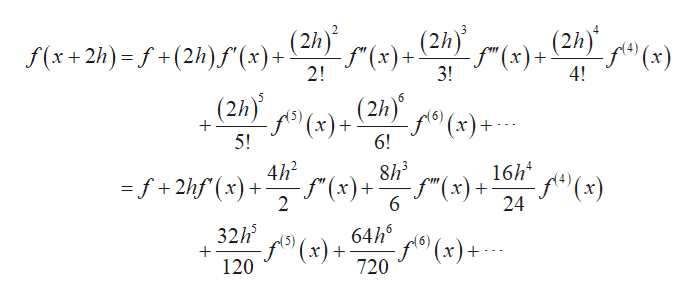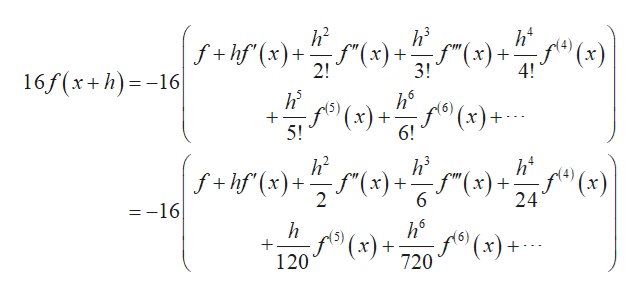-(+2h)+16f (x+h)-30f(x)+165 (x-h)-(-2h)Suppose f"(x)3Show that the error is O(h4)

Questionhelp_outlineImage Transcriptionclose-(+2h)+16f (x+h)-30f(x)+165 (x-h)-(-2h) Suppose f"(x) 3 Show that the error is O(h4) fullscreen
Step 1

It is given that,help_outlineImage Transcriptionclose1 x 2h)+16 f(x+h)-30f(x) +16S(x-h)-f(x-2h)] (x) 12h2 fullscreen
Step 2

Use Taylor’s series to compute f(x+2h) as follows.help_outlineImage Transcriptionclose(2h)(x) (2h) 2 (2h) -S" (х)+ 2! f(x+2h)- f+(2h)f (x)+ (2h) f(x)+ 3! 4! (2h) f(x)+ 6! (x)+ + 5! 16h f(x) 24 8h3 4h2 f"(x ) )+ f"(x) =f+2hf(x + 6 2 64hx)+ 32h f(x)+ 720 + 120 fullscreen
Step 3

Compute 16(f(x+h)) as foll...help_outlineImage Transcriptioncloseh2 f+hf(x) 2! h's h2 4 f"(x)+ -f"(x)+ 3! 4! 16f(x+h)16 5 6 x)+ 5! 6! h2 h's ht f"(x)+ 24 f+hf (x)+x)+f"(x) 2 6 =-16 h6 f(x)+ 720 5 120 fullscreen

Want to see the full answer?

See Solution

Want to see this answer and more?

Our solutions are written by experts, many with advanced degrees, and available 24/7

See Solution
Tagged in

Math